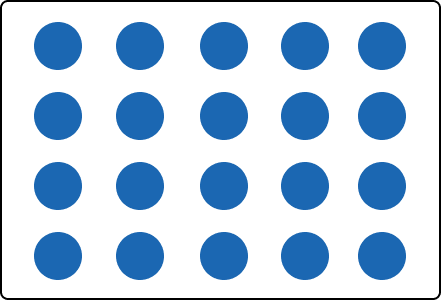# Equal Groups – Definition with Examples

Home » Math-Vocabluary » Equal Groups – Definition with Examples

## What are Equal Groups?

Two groups are called equal groups if they have the same number of objects. So, in equal groups, equal number of objects or things are grouped.

Example: If there are 3 boxes and you put 5 candies in each box, then each box will have the same number of candies. Here, we say that there are 3 equal groups with 5 candies in each group.

## Equal Groups Definition

Groups that have the same number of objects are known as equal groups in math.

For example, look at this picture:

There are 6 stars in each group. So, these are equal groups.

We can write this as: 4 groups of 6 stars each.

## Equal Groups in Real Life

We can find many real life examples where equal groups are used. For example, any two packets of cookies of the same brand and same size have the same number of cookies.A few more examples are shown below:

## Equal Groups as Repeated Addition

If we have multiple equal groups, then we can use repeated addition to find the total number of objects. Let’s see how we can do that.

There are 4 groups, each having 6 stars. So, if we add the number 6 four times, we can find the total number of stars.

So, total number of stars $= 6 + 6 + 6 + 6 = 24$ stars.

## Equal Groups as Multiplication Sentence

There is another way of finding the total number of objects arranged in equal groups. It is multiplication. We can represent equal groups as a multiplication expression and then solve it to find the answer.

Let’s consider the same example of equal groups of stars.

So, the total number of stars $= 4 \times 6 = 24$ stars.

## Equal Groups as Division Sentence

Division means distributing something into equal groups. So, if we are given equal groups, we can express them as a division expression. The quotient represents the number of groups or the group size. That is,

Total Number $\div$ Group Size = Number of Groups

Total Number $\div$ Number of Groups = Group Size

## A Case of Equal Groups

Eddie visits a cookie store to purchase some cookies for guests. He places an order for 24 cookies on the counter. The cookies are packed in groups of 8. Eddie starts to figure out the answer to the question, “How many packets of cookies will he get?”

Well, Eddie has learnt in his math class that models come handy in these sorts of situations. He creates a model in his mind that helps him find out how many equal groups he can make.

The storekeeper gave Eddie 3 packets. So, we can say that Eddie did his mind calculations correctly.

## Equal Groups as Arrays

There is yet another way of representing groups that are equal and it is more organized. Consider the same example of 4 equal groups of stars. This time, arrange the stars in rows and columns.

This arrangement of the objects in rows and columns is known arrays. Multiplying the number of rows and columns in the given array gives the total number of objects.

So, the total number of stars = rows × columns $= 4 \times 6 = 24$ stars.

## Solved Examples

1. James places some gummies on 3 plates as shown below. Is this equal grouping?

Solution:

Yes. This shows equal grouping because James placed an equal number of gummies on each plate, that is, 2 gummies per plate.

1. Suppose you have 20 flowers placed equally in 5 vases. Draw an equal group model that represents this situation.

Solution:

20 flowers can be arranged equally in 5 vases by placing 4 flowers in each vase.

1. Express this array as equal grouping in 2 different ways—equal rows and equal columns.

Solution:

Equal columns: There are 7 columns of 3 counters each.

## Practice Problems

1

### There are 7 groups of 4 apples each. Choose the repeated addition expression that represents this equal grouping.

$7 + 4$
$4 + 4$
$7 + 7 + 7 + 7$
$4 + 4 + 4 + 4 + 4 + 4 + 4$
CorrectIncorrect
Correct answer is: $4 + 4 + 4 + 4 + 4 + 4 + 4$
7 groups of 4 means we must add 4 repeatedly for 7 times. That is, $4 + 4 + 4 + 4 + 4 + 4 + 4$
2

### Which multiplication expression describes this array?$4 \times 4$
$4 \times 5$
$4 + 5$
$5 + 5$
CorrectIncorrect
Correct answer is: $4 \times 5$
There are 4 rows of 5 counters. So, the multiplication expression representing this array would be $4 \times 5$.
3

### Jose buys 6 packs of 5 highlighters. How many highlighters does he buy in all?

30
36
25
11
CorrectIncorrect
Hence, the total number of highlighters $= 5 + 5 + 5 + 5 + 5 + 5 = 30$ highlighters.
We can find the total number of items in equal groups by repeated addition or by multiplication. If there are 4 groups of 6 apples then the total number of apples $= 6 + 6 + 6 + 6 = 24$ or $4 \times 6 = 24$ apples.